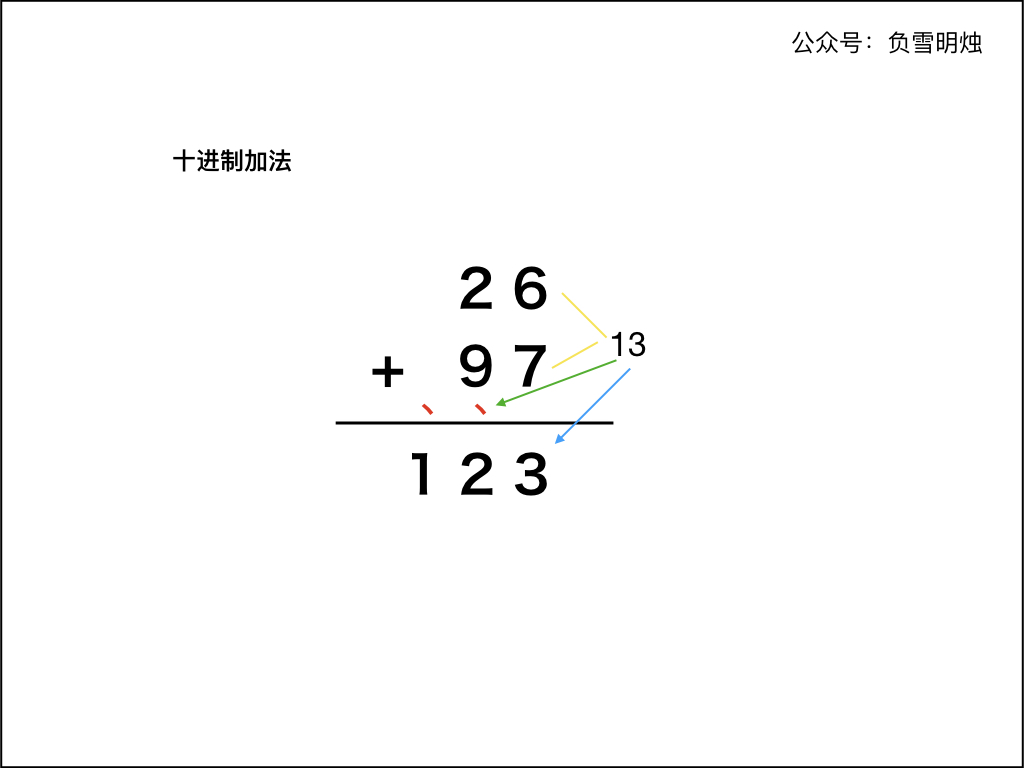# 445. Add Two Numbers II 两数相加 II

• 作者： 负雪明烛
• id： fuxuemingzhu
• 个人博客： http://fuxuemingzhu.cn/
• 个人公众号：负雪明烛
• 本文关键词：两数相加，链表，求加法，题解，leetcode, 力扣，python, c++, java

@TOC

# # 题目描述

You are given two non-empty linked lists representing two non-negative integers. The most significant digit comes first and each of their nodes contain a single digit. Add the two numbers and return it as a linked list.

You may assume the two numbers do not contain any leading zero, except the number 0 itself.

What if you cannot modify the input lists? In other words, reversing the lists is not allowed.

Example:

Input: (7 -> 2 -> 4 -> 3) + (5 -> 6 -> 4)
Output: 7 -> 8 -> 0 -> 7


# # 解题方法

## # 前言

• 两个字符串形式的数字相加（第 415 题）
• 两个链表形式的数字相加（第 2 、445、369 题）
• 数组形式的数字相加（第 66 、989题）
• 两个二进制形式的数字相加（第 67 题）

## # 十进制加法1. 两个「加数」的右端对齐；
2. 从最右侧开始，从右向左依次计算对应的两位数字的和，如果有进位需要加上进位。如果和大于等于 10，则把和的个位数字计入结果，并向前面进位；
3. 重复步骤 2；
4. 当两个「加数」的每个位置都计算完成，如果最后仍有进位，需要把进位数字保留到计算结果中。

## # 在实现中需要注意的有：

1. 不可以把链表/字符串表示的「加数」先转化成 int 型数字再求和，因为可能溢出
2. 两个「加数」的字符串长度可能不同；
3. 在最后，如果进位 carry 不为 0，那么最后需要计算进位
4. 注意 结果数字 是否为低位结果在前，根据题目要求判断最后是否要反转结果

## # 方法：栈 + 循环

### # 步骤：

1. 先对两个链表分别遍历放到栈中；
2. 从栈中分别弹出栈顶数字 adder1adder2，计算 adder1adder2 之和，再加上进位 carry，得到当前位置的和 sum
1. 如果 sum >= 10 ，那么进位 carry = 1 ，当前位设置为 sum - 10
2. 如果 sum < 10，那么进位 carry = 0，当前位设置为 sum
3. 设置新链表节点，其值为 sum逆序拼接成链表即可。

### # 代码中的巧妙之处：

1. while (!st1.empty() || !st2.empty() || carry > 0) 含义：
1. 栈 1 和 栈 2 只要有一个没遍历完，那么就继续遍历；
2. 如果栈 1 和 栈 2 都遍历完了，但是最后留下的进位 carry != 0，那么需要把进位也保留到结果中。
2. 取栈顶元素的时候，如果栈 1 或 栈 2 已经遍历完了（即 或者 ），则认为 当前的加数是
3. 逆序拼接链表的方法：先定义了一个哑结点dummy ，然后每次把新构建的链表结点放到 dummydummy->next 之间，最后返回结果是 dummy->next

Java 代码如下：

/**
* Definition for singly-linked list.
* public class ListNode {
*     int val;
*     ListNode next;
*     ListNode() {}
*     ListNode(int val) { this.val = val; }
*     ListNode(int val, ListNode next) { this.val = val; this.next = next; }
* }
*/
class Solution {
public ListNode addTwoNumbers(ListNode l1, ListNode l2) {
Stack<Integer> st1 = new Stack();
Stack<Integer> st2 = new Stack();
while (l1 != null) {
st1.push(l1.val);
l1 = l1.next;
}
while (l2 != null) {
st2.push(l2.val);
l2 = l2.next;
}
int carry = 0;
ListNode dummy = new ListNode(0);
while (!st1.empty() || !st2.empty() || carry > 0) {
int adder1 = st1.empty() ? 0 : st1.pop();
int adder2 = st2.empty() ? 0 : st2.pop();
int sum = adder1 + adder2 + carry;
carry = sum >= 10 ? 1 : 0;
sum = sum >= 10 ? sum - 10 : sum;
ListNode cur = new ListNode(sum);
cur.next = dummy.next;
dummy.next = cur;
}
return dummy.next;
}
}


C++ 代码如下：

/**
* Definition for singly-linked list.
* struct ListNode {
*     int val;
*     ListNode *next;
*     ListNode() : val(0), next(nullptr) {}
*     ListNode(int x) : val(x), next(nullptr) {}
*     ListNode(int x, ListNode *next) : val(x), next(next) {}
* };
*/
class Solution {
public:
ListNode* addTwoNumbers(ListNode* l1, ListNode* l2) {
stack<int> st1;
stack<int> st2;
while (l1) {
st1.push(l1->val);
l1 = l1->next;
}
while (l2) {
st2.push(l2->val);
l2 = l2->next;
}
int carry = 0;
ListNode* dummy = new ListNode(0);
while (!st1.empty() || !st2.empty() || carry > 0) {
int adder1 = 0;
int adder2 = 0;
if (!st1.empty()) {
st1.pop();
}
if (!st2.empty()) {
st2.pop();
}
int sum = adder1 + adder2 + carry;
carry = sum >= 10 ? 1 : 0;
sum = sum >= 10 ? sum - 10 : sum;
ListNode* cur = new ListNode(sum);
cur->next = dummy->next;
dummy->next = cur;
}
return dummy->next;
}
};


Python 代码如下：

# Definition for singly-linked list.
# class ListNode(object):
#     def __init__(self, val=0, next=None):
#         self.val = val
#         self.next = next
class Solution(object):
def addTwoNumbers(self, l1, l2):
st1 = []
st2 = []
while l1:
st1.append(l1.val)
l1 = l1.next
while l2:
st2.append(l2.val)
l2 = l2.next
carry = 0
dummy = ListNode(0)
while st1 or st2 or carry:
adder1 = st1.pop() if st1 else 0
adder2 = st2.pop() if st2 else 0
sum = adder1 + adder2 + carry
carry = 1 if sum >= 10 else 0
sum = sum - 10 if sum >= 10 else sum
cur = ListNode(sum)
cur.next = dummy.next
dummy.next = cur
return dummy.next


• 时间复杂度： 分别是链表 ab 的长度；
• 空间复杂度：，只使用了常数的空间。

# # 总结

1. 求加法」系列题目都不难，其实就是 「列竖式」 计算。
2. 需要注意的是：
1. while循环结束条件；
2. 遍历两个「加数」不要越界；
3. 进位。
4. 链表在插入的时候如何进行反转

# # 日期

2018 年 2 月 26 日 2019 年 2 月 22 日 —— 这周结束了 2021 年 10 月 31 日 —— 把求加法系列的题目整理完了